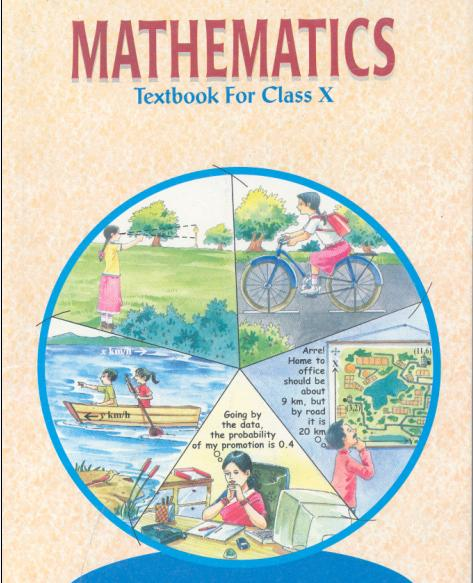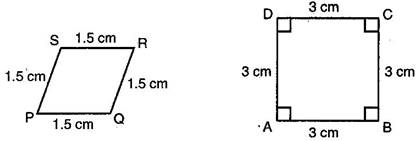# NCERT Solutions for Class 10 Maths Exercise 6.1## myCBSEguide App

CBSE, NCERT, JEE Main, NEET-UG, NDA, Exam Papers, Question Bank, NCERT Solutions, Exemplars, Revision Notes, Free Videos, MCQ Tests & more.

NCERT solutions for Maths Triangles## NCERT Solutions for Class 10 Maths Triangles

1. Fill in the blanks using the correct word given in brackets:

(i) All circles are _______________. (congruent, similar)

(ii) All squares are _______________. (similar, congruent)

(iii) All _______________ triangles are similar. (isosceles, equilateral)

(iv) Two polygons of the same number of sides are similar, if (a) their corresponding angles are _______________ and (b) their corresponding sides are _______________. (equal, proportional)

Ans. (i) similar

(ii) similar

(iii) equilateral

(iv) equal, proportional

2. Give two different examples of pair of:

(i) similar figures

(ii) non-similar figures

Ans. (i) Two different examples of pair of similar figures are:

(a) Any two rectangles

(b) Any two squares

(ii) Two different examples of pair of non-similar figures are:

(a) A scalene and an equilateral triangle

(b) An equilateral triangle and a right angled triangle

3. State whether the following quadrilaterals are similar or not:Ans. On looking at the given figures of the quadrilaterals, we can say that they are not similar because their angles are not equal.

## NCERT Solutions for Class 10 Maths Exercise 6.1

NCERT Solutions Class 10 Maths PDF (Download) Free from myCBSEguide app and myCBSEguide website. Ncert solution class 10 Maths includes text book solutions from Mathematics Book. NCERT Solutions for CBSE Class 10 Maths have total 15 chapters. 10 Maths NCERT Solutions in PDF for free Download on our website. Ncert Maths class 10 solutions PDF and Maths ncert class 10 PDF solutions with latest modifications and as per the latest CBSE syllabus are only available in myCBSEguide.

## CBSE app for Class 10

To download NCERT Solutions for Class 10 Maths, Computer Science, Home Science,Hindi ,English, Social Science do check myCBSEguide app or website. myCBSEguide provides sample papers with solution, test papers for chapter-wise practice, NCERT solutions, NCERT Exemplar solutions, quick revision notes for ready reference, CBSE guess papers and CBSE important question papers. Sample Paper all are made available through the best app for CBSE### 1 thought on “NCERT Solutions for Class 10 Maths Exercise 6.1”

1. Good for student